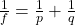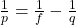A camera lens used for taking close-up photographs has a focal length of 22.0 mm. The farthest it can be placed from the film is 32.9 mm. Wh

Question

A camera lens used for taking close-up photographs has a focal length of 22.0 mm. The farthest it can be placed from the film is 32.9 mm. What is the closest object that can be photographed

in progress 0
6 months 2021-07-31T17:28:15+00:00 1 Answers 3 views 0

p = 6.64 cm

Explanation:

For this exercise we use the equation of the constructorwhere f is the focal length, p and q are the distance to the object and the image, respectively

They tell us the focal length f = 2.2 cm and that the image as far as it can go is q = 3.29 cm, let’s find the position of the object that creates this image1 / p = 1 / 2.2 – 1/3.29

1 / p = 0.15059

p = 6.64 cm

therefore the farthest distance from the object is 6.64 c